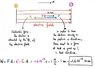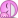# Work and electric field

• JulienB
The sign of the work done by the electric field is positive, and the sign of the work that must be performed to move the electron is also positive. This means that work is done on the electron by the electric field.f

## Homework Statement

Hi everybody! Here I am with another problem of electromagnetism, I'd like to make sure I understand it properly!

An electron is moved 1 meter towards an homogeneous electric field of strength 1N/C. How big is the work done for this motion?

## Homework Equations

$$F = q \cdot E$$

## The Attempt at a Solution

Here is what I've done:

$$W = F \cdot d = q \cdot E \cdot d = -1.6 \cdot 10^{-19} C \cdot 1 \frac{N}{C} \cdot 1m = -1.6 \cdot 10^{-19} N \cdot m$$

It seems a little too easy... Is that correct?

Thanks a lot for your help.

Julien.

You have to be careful about the signs.

The direction of an electric field at a given point is, by convention, the direction that a positive charge at that point will be accelerated. If the electron is moved toward that field, would it be in the direction of, or opposite to, the direction of the electric field? "Toward" is somewhat ambiguous, but I expect that it means opposite to the direction of the field. If so, the displacement direction is opposite to the direction of the field. What does that do to the sign of your answer? Does this mean that work is done on or by the electron?

AM

@Andrew Mason Hi and thank you for your answer. I understand what you're saying, I realized that myself after posting. The electron is attracted by the + side of the electric field, but is moving towards the - (because of an unspecified force). That means the work is done on the electron by this unspecified force. Is that right?

The expression for work that you gave is the work done by the electric force. If the displacement vector is opposite in sign to the field vector what is the sign of the result (ie. the work done by the electric force)?

AM

Ok. You should show us why you think it is positive.

What does that tell you about whether positive work is done on the electron by the electric field? Another way of looking at this is: does the electron's kinetic energy increase or decrease as a result of the work done?

AM

That work being positive means that work is done on the electron by the electric field. But, wait, I read again your last post where you say "If the displacement vector is opposite in sign to the field vector (...)". I thought the displacement vector was showing in the same direction as the field?? I've attached a drawing explaining how I represent myself the situation, maybe that helps!

Julien.

#### Attachments

•image.jpeg
38.1 KB · Views: 301

That work being positive means that work is done on the electron by the electric field. But, wait, I read again your last post where you say "If the displacement vector is opposite in sign to the field vector (...)". I thought the displacement vector was showing in the same direction as the field?? I've attached a drawing explaining how I represent myself the situation, maybe that helps!
Perhaps you could give us the exact wording of the question. I was not sure what was meant by "toward the electric field" since it is a uniform field. So "toward" is a bit ambiguous. I suggested that this meant that the motion was opposite to the direction of the field.

AM

@Andrew Mason Ah yes, I guess my wording was wrong. Sorry for that, I translated the problem from German to English, and none of them is my mother languageI try another translation, more literal:
"How big is the work that must be performed in order to move an electron for a distance of 1 meter in the positive x-direction in a homogeneous electric field of strength 1N/C pointing in the positive x-direction?"

I guess at the time I posted the problem, I did not properly understand the question myself hence the poor translation...My apologiesJulien.

@Andrew Mason Ah yes, I guess my wording was wrong. Sorry for that, I translated the problem from German to English, and none of them is my mother languageI try another translation, more literal:
"How big is the work that must be performed in order to move an electron for a distance of 1 meter in the positive x-direction in a homogeneous electric field of strength 1N/C pointing in the positive x-direction?"

I guess at the time I posted the problem, I did not properly understand the question myself hence the poor translation...My apologiesJulien.
OK. That is clear now. The dot product ##\vec{E} \cdot \vec{d}## is positive. So what is the sign of the work done by the field? What is the sign of the work that must be performed to move the electron?

AM

So I think the work done by the field is negative and that the work that must be performed to move the electron is positive. Is that right?

Julien.

@Andrew Mason
So I think the work done by the field is negative and that the work that must be performed to move the electron is positive. Is that right?
Correct!

AM

•JulienB